Function Repository Resource:

# PartialOrderGraphQ

Test whether a graph is a partial order, that is, reflexive, antisymmetric and transitive

Contributed by: Wolfram Staff (original content by Sriram V. Pemmaraju and Steven S. Skiena)
 ResourceFunction["PartialOrderGraphQ"][g] yields True if the binary relation defined by edges of the graph g is a partial order and False otherwise.

## Details and Options

The binary relation defined by edges of the graph is a partial order if it is transitive, reflexive and antisymmetric.

## Examples

### Basic Examples (2)

The graph representing the divisibility relation between integers is partial order since the relation is reflexive (each integer divides itself), antisymmetric (n cannot divide m if n>m) and transitive (as n|m implies m=kn for some integer k, so m|l implies n|l):

 In:=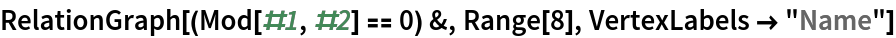Out=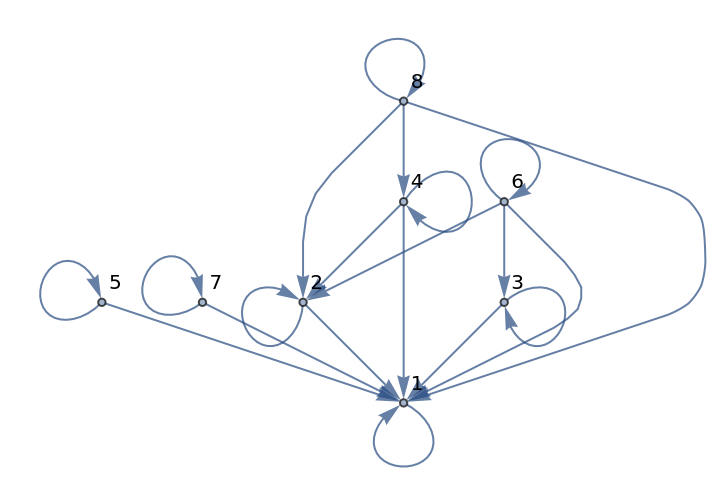In:=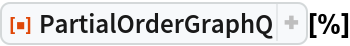Out=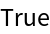A graph formed by selecting ordered tuples is a partial order:

 In:=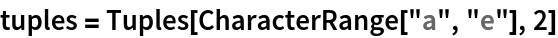Out=In:=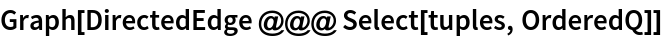Out=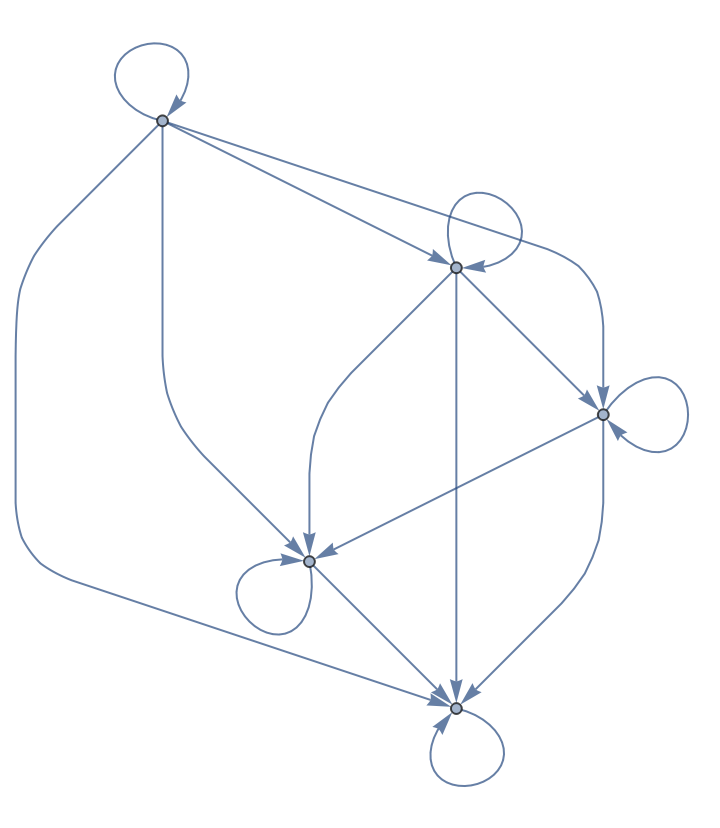In:=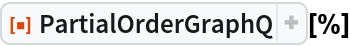Out=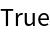On the other hand, a similar graph constructed from the symmetric set of the tuples is not:

 In:=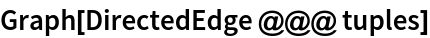Out=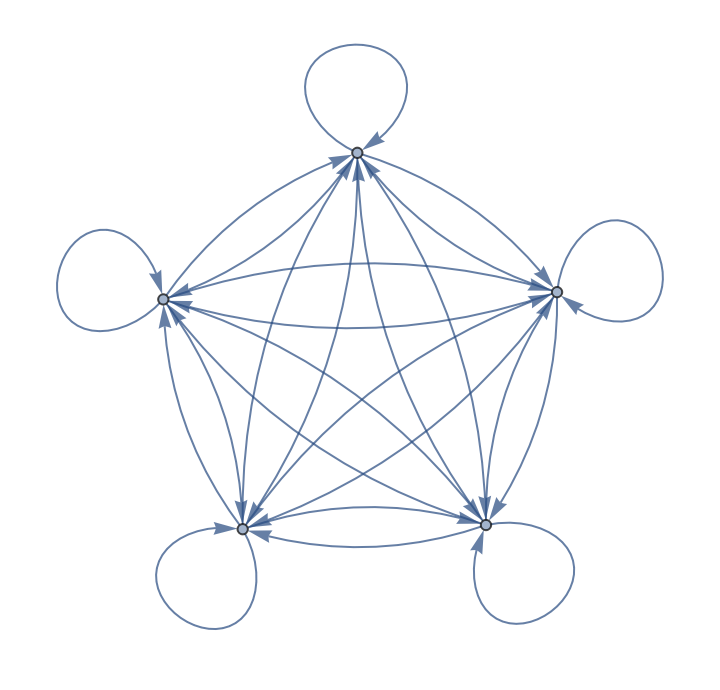In:=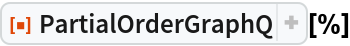Out=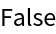## Version History

• 1.0.0 – 01 July 2020